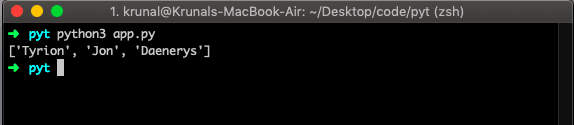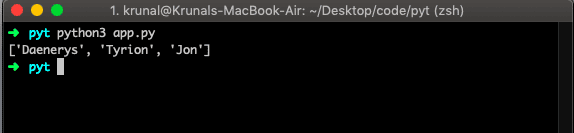# Python List sort: The Complete Guide

## Python List Sort

Python list sort() is an inbuilt function that sorts the list ascending by default. The sort() method sorts the elements of a given list in the specific order, either Ascending or Descending. You can also make the function to decide the sorting criteria.  The sort() method doesn’t return any value. Instead, it changes the original list.

### Syntax

The syntax of the sort() method is the following.

`list.sort(reverse=True|False, key=myFunc)`

### Parameters

The reverse parameter value is optional, and reverse=True will sort the list descending. The default is reverse=False.

The key parameter value is optional, and it is the function to specify the sorting criteria.

### Return Value

The sort() method doesn’t return any value. First, it changes the original list.

If you want the original list, use sorted().

See the following example.

## How to Sort in Ascending Order

Let us see the by default sorting on the list.

```# app.py

GoT = ['Jon', 'Tyrion', 'Daenerys']
GoT.sort()
print (GoT)```

See the output.## How to Sort in Descending Order

Now to arrange descending order, we need to add reverse=True in the sort() method’s parameter.

```# app.py

GoT1 = ['Jon', 'Tyrion', 'Daenerys']
GoT1.sort(reverse=True)
print (GoT1)```

See the output.## How to Sort the list using the key

Let us take function criteria to sort the list. We sort the list based on the length of the string and sort the descending order based on the length.

```# app.py

def criteria(item):
return len(item)

GoT2 = ['Jon', 'Tyrion', 'Daenerys']
GoT2.sort(reverse=True, key=criteria)
print (GoT2)```

See the output.## How to sort the list of lists

If we make a list of lists, then the list will be sorted based on what the first item of every list is.

See the following code.

```city_list = [['Omaha', 'Lincoln', 'Norfolk'],
['LA', 'San Diego', 'San Francisco'],
['Las Vegas', 'Carson City', 'Laughlin']]

city_list.sort()
print(city_list)
```

#### Output

`[['LA', 'San Diego', 'San Francisco'], ['Las Vegas', 'Carson City', 'Laughlin'], ['Omaha', 'Lincoln', 'Norfolk']]`

You can see that the first two lists starting with La, but the first LA string is the whole upper case, so it takes precedence. Then Omaha is obvious last.

## How to Sort a List of Lists for a Specific Column/Index

Let’s say that you want to sort the list of lists based on the population column (which has the index of ‘1’). In that case, you may use lambda to sort the list of lists for a specific index.

See the following code.

```city_list = [['Omaha', 11],
['LA', 30],
['Las Vegas', 20]]

city_list.sort(key=lambda i: i)
print(city_list)
```

#### Output

`[['Omaha', 11], ['Las Vegas', 20], ['LA', 30]]`

## How can you sort the list of strings in a case insensitive manner

In List.sort() function, the key parameter specifies the function that will be called on each list item before making the comparisons.

This is indeed very helpful because now we can pass the str.lower as the key parameter to a sort() function.

And this will instruct the sort function to perform comparisons between the all-lowercase versions of the strings, which is exactly what we want!

See the following code.

```data_list = ['Asset', 'share', 'Bond', 'gold', 'Cash']

data_list.sort(key=str.lower)
print(data_list)
```

#### Output

`['Asset', 'Bond', 'Cash', 'gold', 'share']`

As you can see, now the sorting is case insensitive.

The key parameter is very robust as it allows us to define our custom sorting function, as we will see later.

## Python sorted()

The sorted() function returns the sorted list of the provided iterable object. You can define ascending or descending order.

Python Strings are sorted alphabetically, and numbers are sorted numerically. Note: You cannot sort the list that contains BOTH string values and numeric values.

The difference between sort() and sorted() function is that sort is the list method that modifies the list in place whereas sorted is an inbuilt function that creates a new list without touching the original one.

See the example of sorted() in Python.

```data_list = [11, 21, 19]

sl = sorted(data_list)
print(sl)
```

#### Output

`[11, 19, 21]`

As you can notice, both list.sort() and sorted sort items in ascending order by default.

That’s it for this tutorial.

This site uses Akismet to reduce spam. Learn how your comment data is processed.